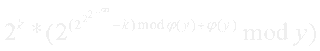### [BZOJ 3884] 上帝与集合的正确用法【欧拉定理/初等数论】

[Description]求值[Solution]

· Solution 1· Solution 2[Code]

· Code 1
#include<cstdio>

typedef long long ll;
const int maxn=10000001;

int phi[maxn]={0,1};
void MakePhiList(){
for(int i=2;i<maxn;i++) if(!phi[i])
for(int j=i;j<maxn;j+=i){
if(!phi[j]) phi[j]=j;
phi[j]=phi[j]/i*(i-1);
}
}

ll pow(ll a,int n,int p){
ll ans=1;
while(n) {
if(n&1) ans=ans*a%p;
a=a*a%p; n>>=1;
}
return ans;
}

ll f(int x){
if(x==1) return 0;
int k=0; while(!(x%2)) x/=2,k++;
return pow(2,(f(phi[x])%phi[x]-k%phi[x]+phi[x])%phi[x]+phi[x],x)<<k;
}

int main(){
MakePhiList();
int kase; scanf("%d",&kase);
while(kase--){
int x; scanf("%d",&x);
printf("%lld\n",f(x));
}
return 0;
}

· Code 2
#include<cmath>
#include<cstdio>

typedef long long ll;

int Phi(int x){
int ans=x;
for(int i=2,lim=sqrt(x)+1;i<lim;i++) if(!(x%i)){
ans-=ans/i;
while(!(x%i)) x/=i;
}
return x>1?ans-ans/x:ans;
}

ll pow(ll a,ll n,ll p){
ll ans=1;
while(n){
if(n&1) ans=ans*a%p;
a=a*a%p; n>>=1;
}
return ans;
}

ll f(int x){
if(x==1) return 0;
int phi=Phi(x);
return pow(2,f(phi)+phi,x);
}

int main(){
int kase; scanf("%d",&kase);
while(kase--){
int x; scanf("%d",&x);
printf("%lld\n",f(x));
}
return 0;
}